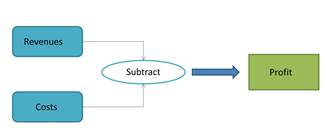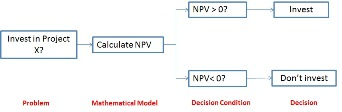# How Mathematical Models are Used in Business

Instructor: Usha Bhakuni

Usha has taught high school level Math and has master's degree in Finance

This lesson will help you understand mathematical models and how they are used in the context of business. You will learn various use-cases of these models in business with the help of relevant examples.

## Mathematical Models

Mathematics can be used to represent real-world situations. The instruments used to represent real world in such a manner are called mathematical models. They help us understand how the real world works.

Most models don't recreate the real world as it is, but they offer a simplified approximation of the real-world situations. A simple mathematical model representing profit calculation is illustrated as -Every mathematical model requires a set of inputs and mathematical functions to generate an output.

## Use of Mathematical Models in Business

There are several ways in which mathematical models are used in the field of business. We will explore some of them here.

### 1. Decision Making

Making decisions is a crucial activity for businesses. It often involves multiple participants with conflicting views. Decision making mathematical models can be of great use here. Such models use input variables and a set of conditions to be fulfilled to help management arrive at a decision.

One of the most common decision-making problem faced by any business is the investment decision, where it must decide whether to invest in a project or not. Businesses often use mathematical models that assess the potential valuation of the project against the investment to be made for making such decisions. Examples of such models are net present value (NPV), internal rate of return (IRR), etc. A simple NPV model can be illustrated as below:### 2. Making predictions

Often businesses have the requirement of predicting certain factors, such as revenue, growth rate, costs, etc. These are usually used in case of new product launch, change in strategy, investment needs, expansion projects, etc. In such cases, predictive mathematical models are used that analyze historical data and use probability distribution as input for predicting the future values.

Regression analysis is one of the most commonly used techniques for predictive models.

Let's understand more with the help of an example of a company that is conceptualizing a new product for kids aged 8-12 years. Before the production, the company would want to understand the potential demand for the product. In doing so, it will require understanding the interests of their target audience. Then, with the help of a predictive model, they can forecast the demand in the future.

### 3. Optimizing

Businesses often need to make optimize certain variables to control costs and ensure maximum efficiency. Such variables might include capacity planning, human resources planning, space planning, route planning, etc. Optimization mathematical models are typically used for such problems. These models often maximize or minimize a quantity by making changes in another variable or a set of variables.

To unlock this lesson you must be a Study.com Member.

### Register to view this lesson

Are you a student or a teacher?

### Unlock Your Education

#### See for yourself why 30 million people use Study.com

##### Become a Study.com member and start learning now.
Back
What teachers are saying about Study.com

### Earning College Credit

Did you know… We have over 160 college courses that prepare you to earn credit by exam that is accepted by over 1,500 colleges and universities. You can test out of the first two years of college and save thousands off your degree. Anyone can earn credit-by-exam regardless of age or education level.